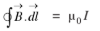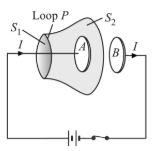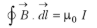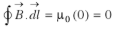# What Is The Inconsistency Of Ampere's Law?

According to Ampere circuital law the line integral of magnetic field B around any closed path is equal to times total current l enclosed by that closed path.Where l is the steady current.

But this equation is logically inconsistent. Consider the charging capacitor, as the charging continues current flows through the connecting wires which changes with time.Consider two surfaces S1 and S2 bounded in the same loop P. When Ampere’s circuital law is applied to the two surfaces S1 and S2 bounded by the same loop P.

For surface S1For surface S2Now the two equations are inconsistent. Although surfaces S1 and S2 belong to the same loop, Ampere law works for surface S1 but it does not work for surface S2. Therefore, Ampere law is ambiguous as it does not provide continuity to current path. This ambiguity has arisen because Ampere’s law is valid for steady currents only. As the capacitor charges the current is changing continuously.